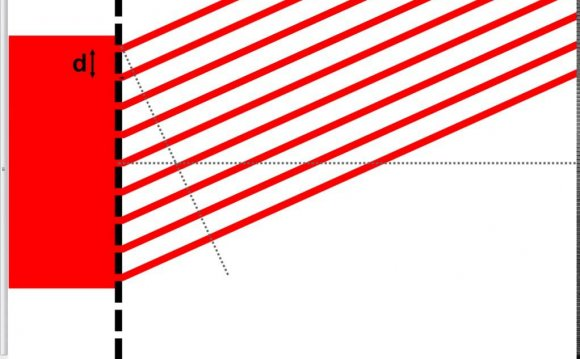# Grating formula

June 2, 2022The grating equation for light diffraction states that for the diffraction pattern that forms when light is passed through an array of regularly spaced openings, that the diffraction order number (n) times the wavelength of the light (λ) is equal to the distance between slits (d) times the sine of the diffraction angle (λ), or:

Because the diffraction angle (θ) is difficult to measure, it is easier to mathematically derive it. If the diffraction pattern is projected onto a screen parallel to and a known distance, h, away from the diffracting grating, the distance from the zeroeth order to the nth order, xn can be measured (see figure 2). Because xn and h are perpendicular to each other, a right triangle is formed with the hypotenuse being the line formed from the diffraction source to the nth order line. Therefore, using trigonometry, θ is equal to the Tan-1 of xn divided by h, or:

 θn=Tan-1(xn/h) (Equation 2)

Which when substituted into equation 1, gives us:

 nλ=dsin(Tan-1(xn/h)) (Equation 3)

Figure 2: Mathematical derivation of angle (θ) of the 1st order diffraction by measurement of the distance from the 0th order to the 1st order lines (x1), and distance from the screen to the grating (h). Note that the lines form a right triangle, and hence trigonometry can be used to calculate θ.

The diffraction technique exploits the fact that coherent laser light constructively interferes with the normal skeletal muscle striation pattern formed by the interdigitation of actin and myosin contractile filaments. These striations, commonly referred to as skeletal muscle A-bands and I-bands behave as a diffraction grating to incident laser light (see Figure 3).

Figure 3: Orderly arrangement of A-bands (thick filaments) and I-bands (thin filaments) within the sarcomeric structure of myofilaments. Light diffracts through the I-band region of the muscle, and the spacing between I-bands is governed by sarcomere length.

Since the spacing of the I-bands is the same as the sarcomere length (since the Z-line is at the center of the I-band, and sarcomere length is from Z-line to Z-line), sarcomere length is then equivalent to d in the grating equation. Thus, given a laser beam of known wavelength, λ, a screen of known distance, h, from the muscle fibers, and a measurement of the distance between the 0th order to the nth diffraction order, xn, one can calculate sarcomere length (SL) with the following equation:

 SL=d=nλ/(sin(Tan-1(xn/h))) (Equation 4)

As one can infer from the equation, increases in sarcomere length would translate to closer distances between diffraction lines.

This technique is a useful tool to determine sarcomere length, both in vivo and in vitro. Examples of in vivo applications include the measurement of sarcomere length changes in fish while they are swimming and intraoperative sarcomere length measurements in humans during reconstructive procedures. Examples of in vitro applications are mechanical studies in which resting sarcomere length needs to be determined or controlled, and changes can be tracked while the muscle bundle or single fiber is activated. Knowledge of a fiber's sarcomere length is important to determine where you are on the length-tension curve and make sure the muscle is at a length which optimal for the tasks it needs to perform.

Source: muscle.ucsd.edu
##### RELATED VIDEOdiffraction grating formula155 Diffraction grating formuladiffraction grating formula example